Next: Bleed Tapping Up: Fluid Section Types: Gases Previous: Fluid Section Types: Gases   Contents

### Orifice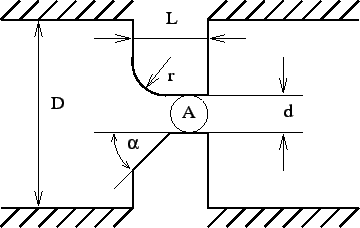Properties: adiabatic, not isentropic, symmetric only if physically symmetric (i.e. same corner radius, corner angle etc.), else directional.

The geometry of the orifice fluid section is shown in Figure 85. The axis of the orifice goes through the center of gravity of the cross section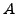and is parallel to the side walls. The orifice is allowed to rotate about an axis parallel to the orifice axis and can be preceded by a swirl generating device such as another orifice, a bleed tapping or a preswirl nozzle.

The orifice element is characterized by an end node well upstream of the smallest section(let's call this position 1) and an end node 2 well downstream of the smallest section (position 2). The smallest section of the gas stream is called position m. This may be smaller thandue to a contraction of the gas and will be written as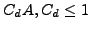.

In between position 1 and m the flow is assumed to be isentropic, consequently

• the mass flow (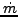) is constant
• the total temperature (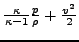) is constant
•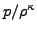is constant
where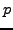is the static pressure. Furthermore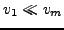is assumed, since the cross section at position 1 is assumed to be large and consequently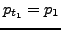,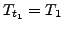.

In between position m and 2 the flow is assumed to be adiabatic, however, all kinetic energy from position m is assumed to be lost due to turbulence. Hence:

• the mass flow () is constant
• the total temperature is constant
•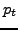at position 2 is equal toat position m.

Combining this leads to the following equation: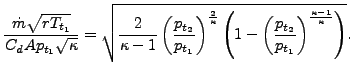(48)

Let us assume that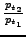is being slowly decreased starting from 1. Then the above equation will result in a steadily increasing mass flow rate up to a maximum at (Figure 86)(49)

after which the mass flow rate starts to decrease again . In reality, the decrease does not happen and the mass flow rate remains constant. Indeed, at maximum corrected flow sonic conditions are reached (so-called critical conditions). For lower values ofthe flow is supersonic, which means that waves cannot travel upstream. Therefore, the information that the pressure ratio has decreased below the critical ratio cannot travel opstream and the critical corrected flow persists throughout. Consequently, for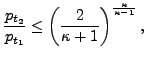(50)

Equation (48) is replaced by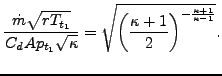(51)

The orifice element is characterized by the following constants (to be specified in that order on the line beneath the *FLUID SECTION card):

• the cross section.
• the orifice diameter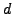(not needed for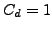).
• the length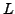(not needed for).
• the inlet corner radius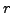(mutually exclusive with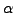; not needed for).
• the inlet corner anglein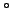(mutually exclusive with; not needed for).
• the orifice-to-upstream pipe diameter ratio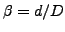(only for TYPE=ORIFICE_PK_MS).
• the rotational velocity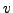, if the orifice is part of a rotating structure (not needed for).
• a reference network element (not needed for).

Depending on the orifice geometry, an inlet corner radius or an inlet corner angle (chamfered inlet) should be selected. They are mutually exclusive. The corrections for a chamfered inlet are taken from .

The last constant, i.e. the number of a reference network element, is necessary in case a rotating structure is preceded by a network element which diverts the upstream air velocity from the axial (i.e. in the direction of the axis of the orifice) direction (such as a preswirl nozzle). In that case, the rotational velocity of the orifice has to be corrected by the circumferential component of the velocity at the exit of the preceding element.

Notice that the only effect of all constants following the cross section is to change the discharge coefficient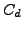. Its calculation can be performed according to different formulas. This is selected by the TYPE parameter:

• TYPE=ORIFICE_CD1 or just TYPE=ORIFICE:.
• TYPE=ORIFICE_MS_MS: Basis formula by McGreehan and Schotsch, rotational correction by McGreehan and Schotsch .
• TYPE=ORIFICE_PK_MS: Basis formula by Parker and Kercher , rotational correction by McGreehan and Schotsch .

Example files: linearnet, vortex1.Next: Bleed Tapping Up: Fluid Section Types: Gases Previous: Fluid Section Types: Gases   Contents
guido dhondt 2018-12-15